# Odd Pie Fights Placing an odd number of

• Slides: 43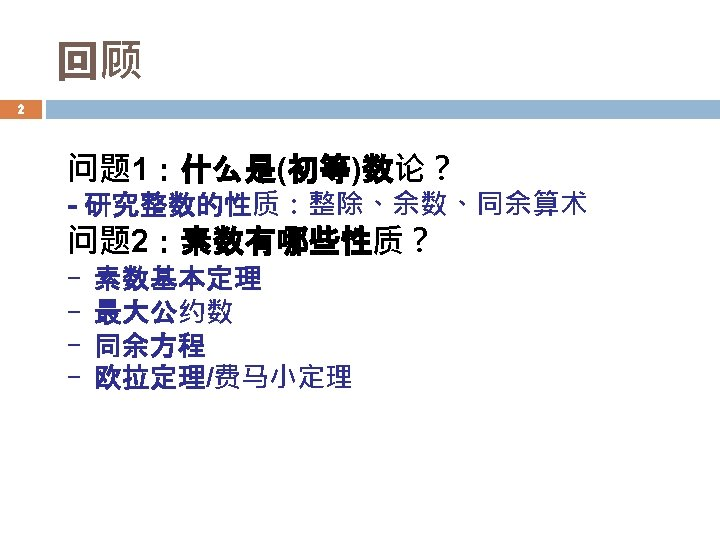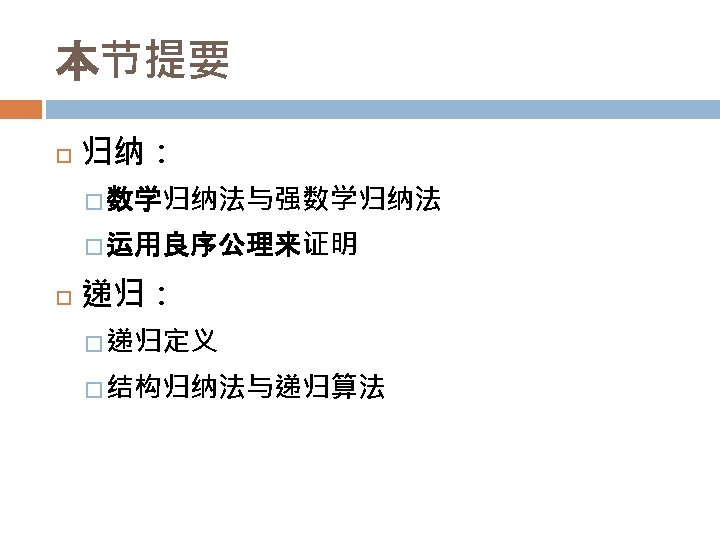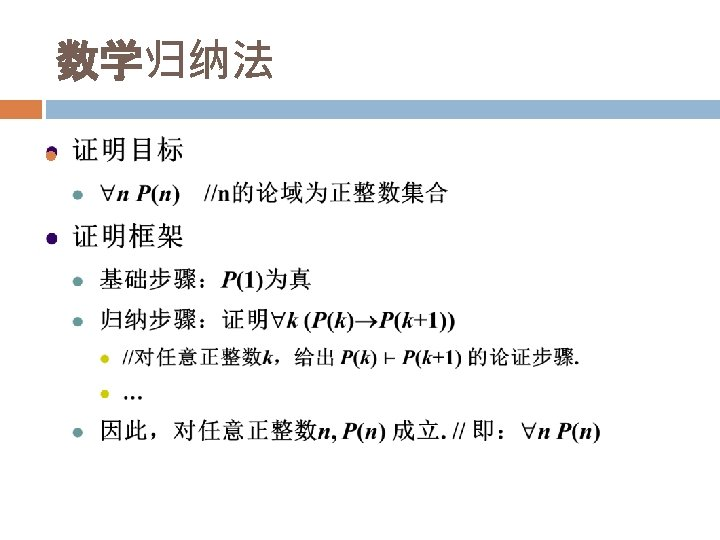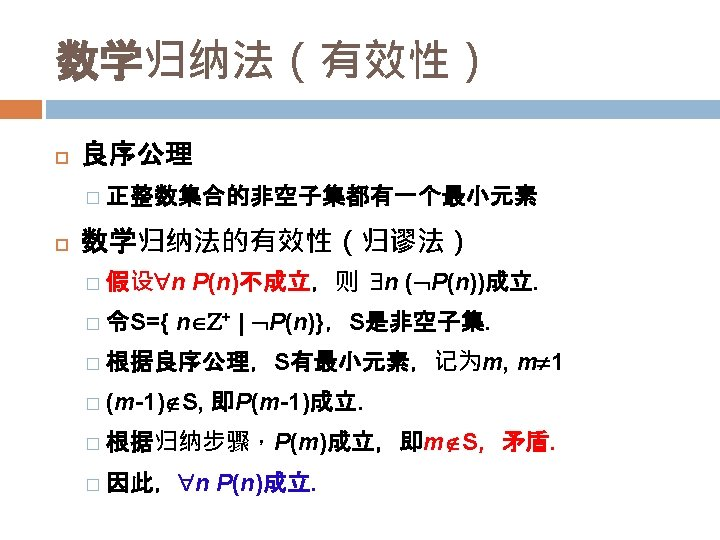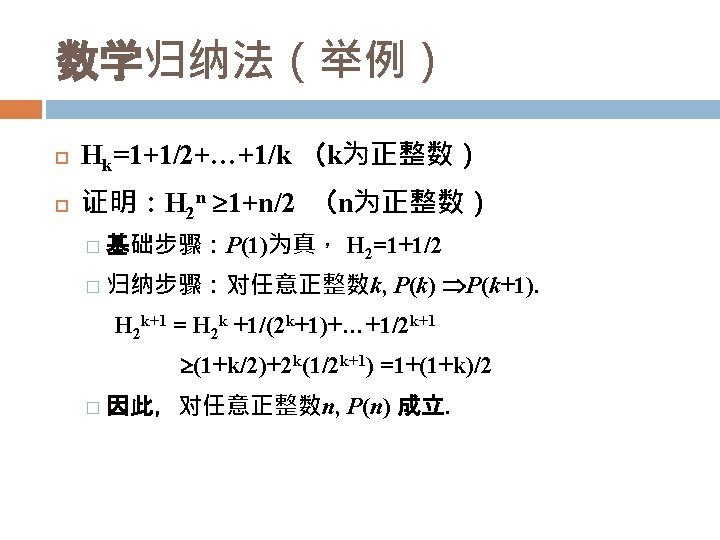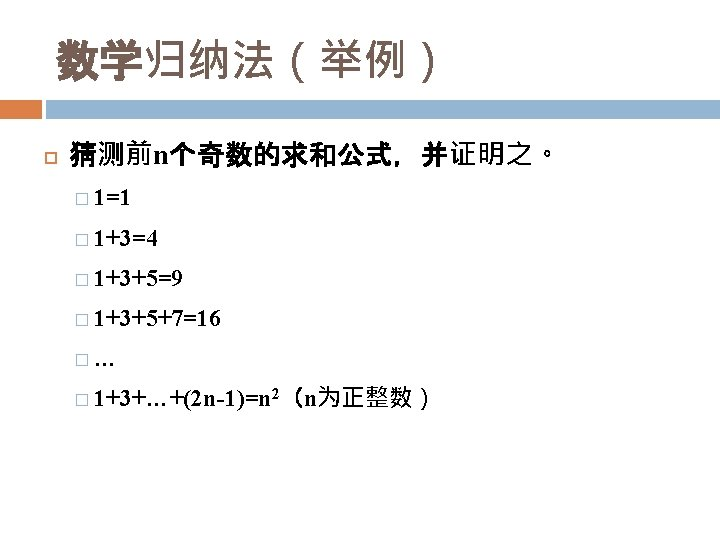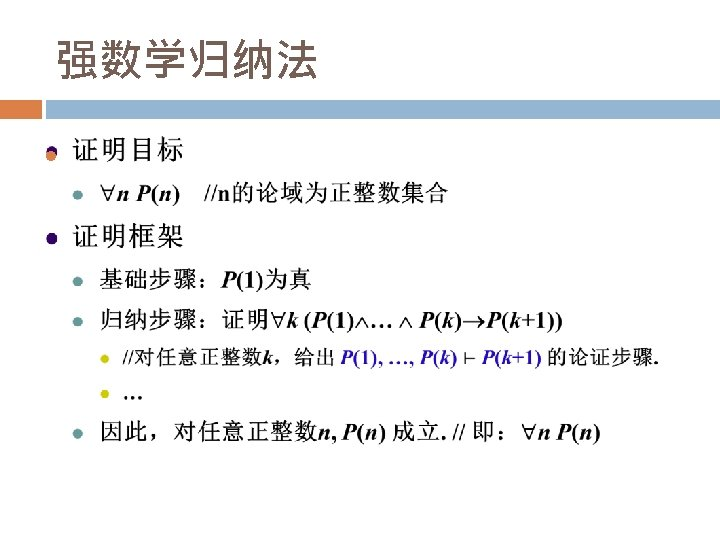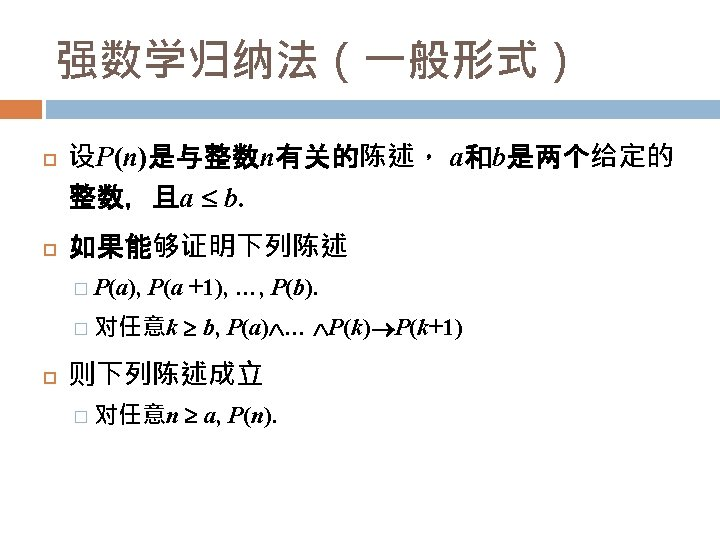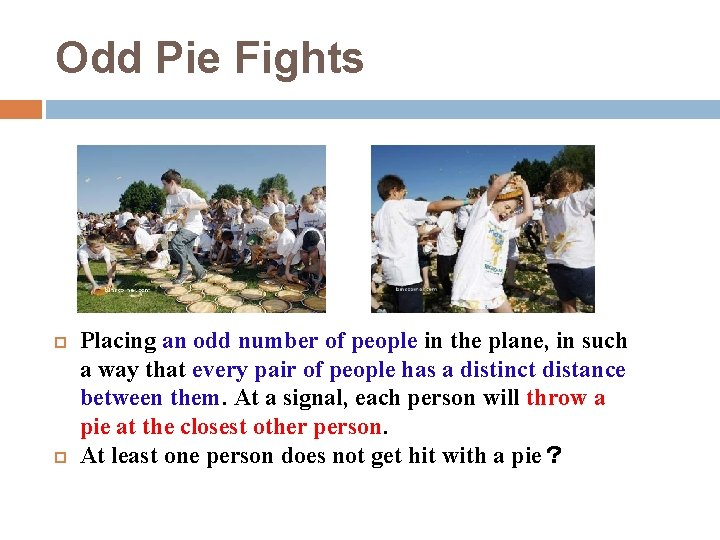Odd Pie Fights Placing an odd number of people in the plane, in such a way that every pair of people has a distinct distance between them. At a signal, each person will throw a pie at the closest other person. At least one person does not get hit with a pie？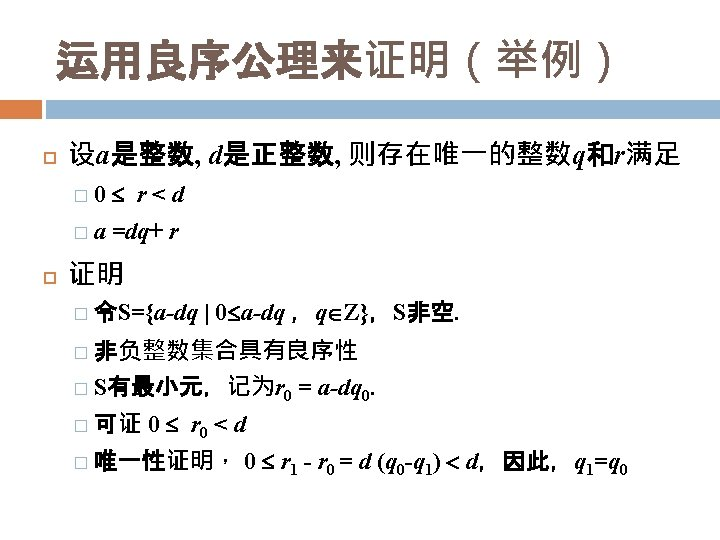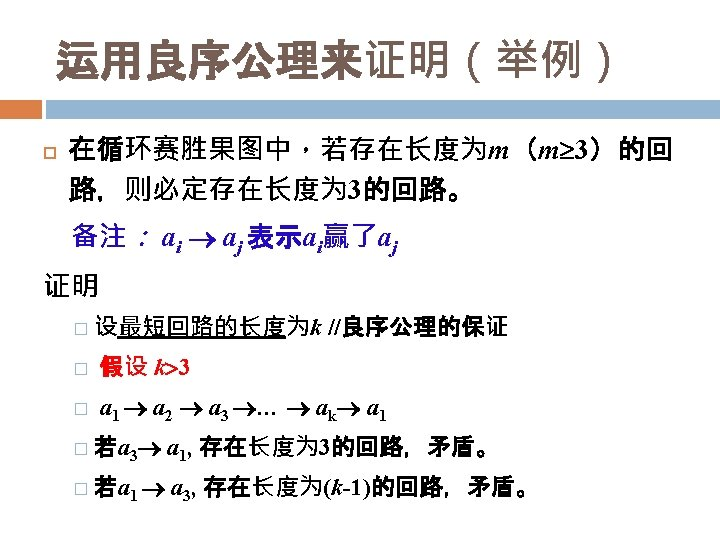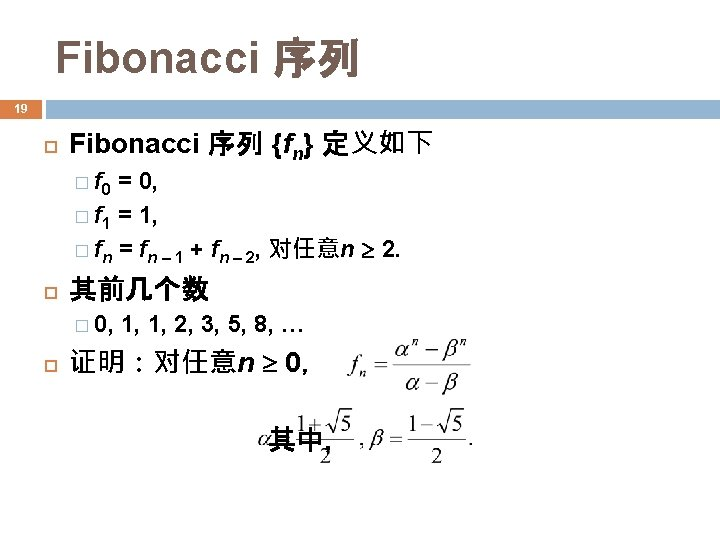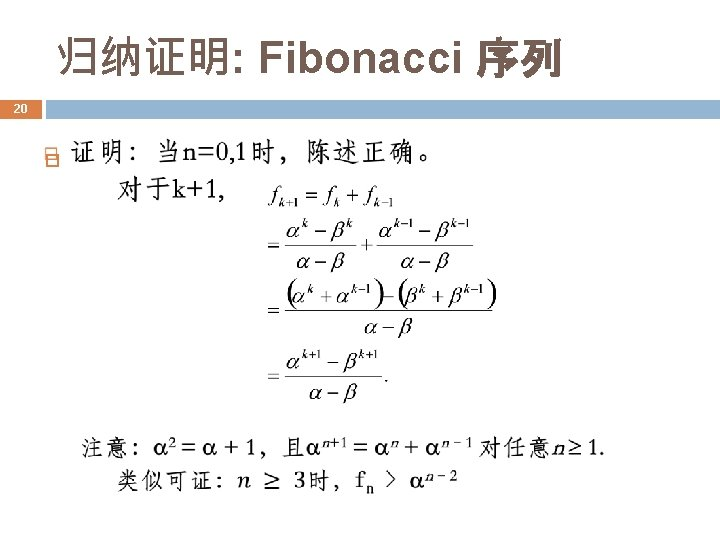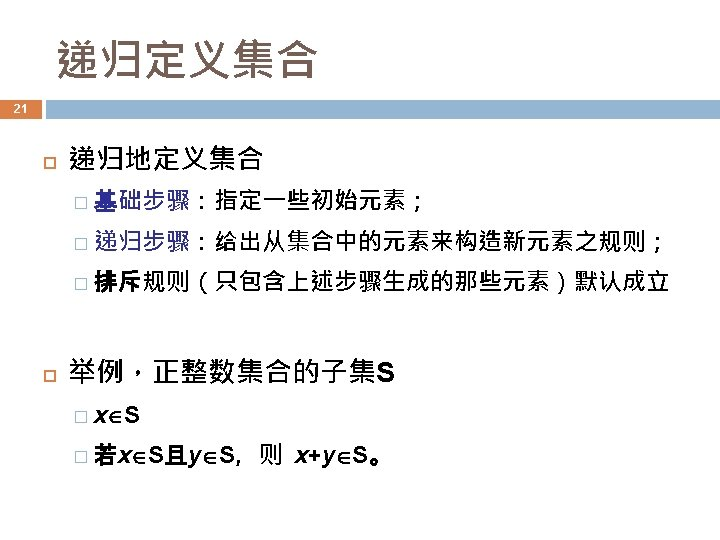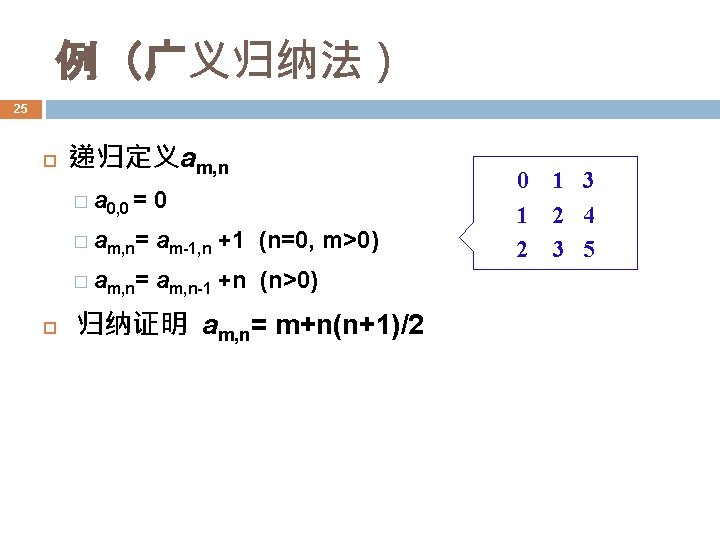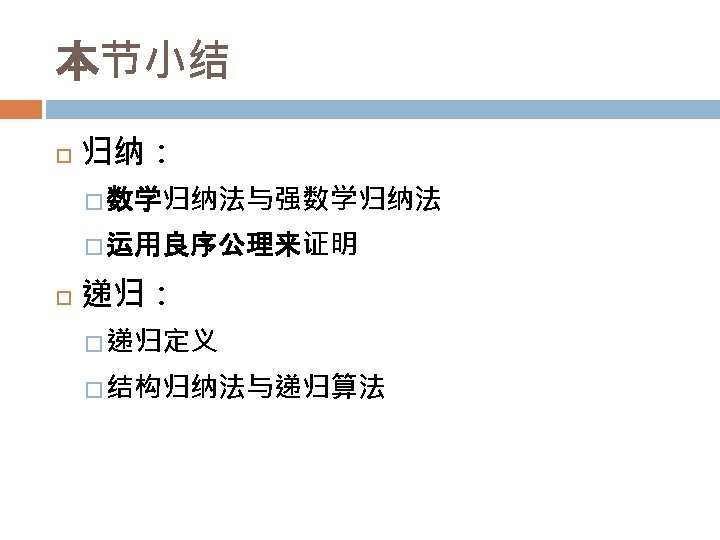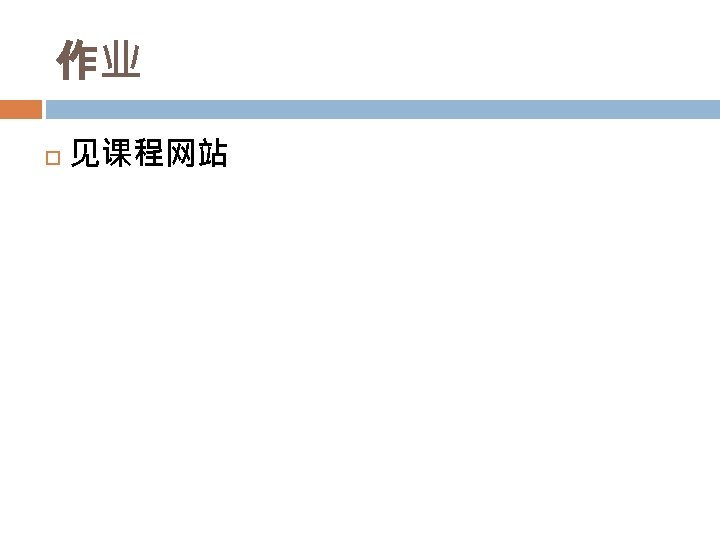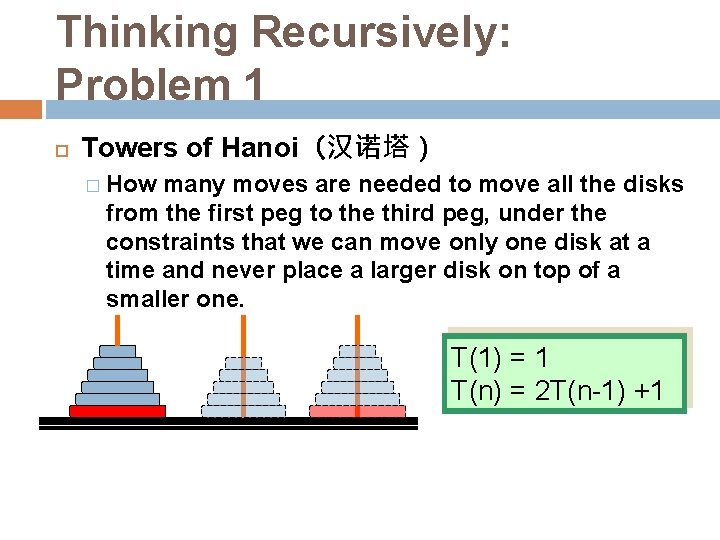Thinking Recursively: Problem 1 Towers of Hanoi（汉诺塔） � How many moves are needed to move all the disks from the first peg to the third peg, under the constraints that we can move only one disk at a time and never place a larger disk on top of a smaller one. T(1) = 1 T(n) = 2 T(n-1) +1Thinking Recursively: Problem 1 Towers of Hanoi（汉诺塔） T(n) = 2 T(n-1) + 1 2 T(n-1) = 4 T(n-2) + 2 4 T(n-2) = 8 T(n-3) + 4 ……. 2 n-2 T(2) = 2 n-1 T(1) + 2 n-2 T(n) = 2 n-1Thinking Recursively: Problem 2 Cutting the plane（平面切割） � How many sections can be generated at most by n straight lines with infinite length. L(0) = 1 L(n) = L(n-1) +n Intersecting all n-1 existing lines to get as most sections as possible Line n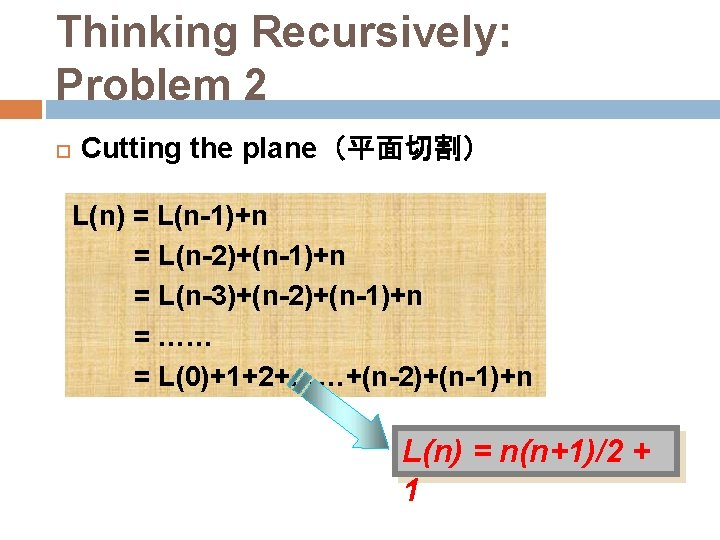Thinking Recursively: Problem 2 Cutting the plane（平面切割） L(n) = L(n-1)+n = L(n-2)+(n-1)+n = L(n-3)+(n-2)+(n-1)+n = …… = L(0)+1+2+……+(n-2)+(n-1)+n L(n) = n(n+1)/2 + 1Thinking Recursively: Problem 3 Josephus Problem：Live or die, it’s a problem! � Legend has it that Josephus wouldn't have lived to become famous without his mathematical talents. During the Jewish Roman war, he was among a band of 41 Jewish rebels trapped in a cave by the Romans. Preferring suicide to capture, the rebels decided to form a circle and, proceeding around it, to kill every third remaining person until no one was left. But Josephus, along with an unindicted co-conspirator, wanted none of this suicide nonsense; so he quickly calculated where he and his friend should stand in We use a simpler the vicious circle. version: “every second. . . ”Thinking Recursively: Problem 3 1 Make a Try: for n=10 10 1 3 9 2 3 9 8 7 6 5 7 4 5 1 3 9 7 5 survivor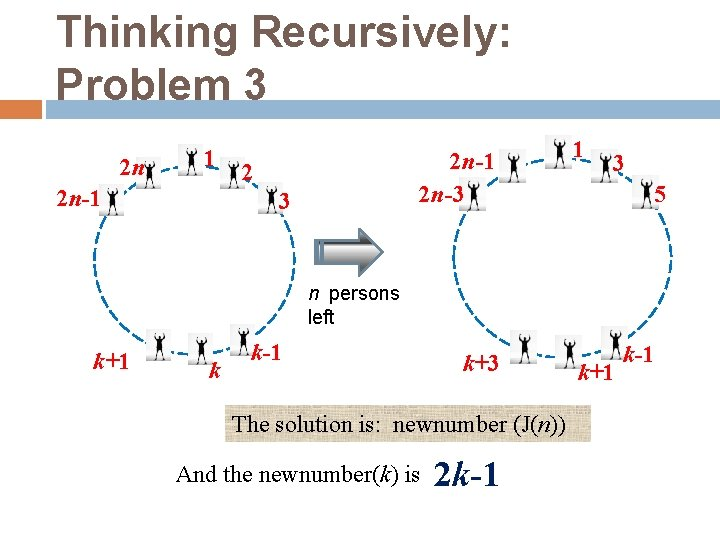Thinking Recursively: Problem 3 2 n 1 2 n-1 2 n-3 2 3 1 3 5 n persons left k+1 k k-1 k+3 The solution is: newnumber (J(n)) And the newnumber(k) is. . . 2 k-1 k+1 k-1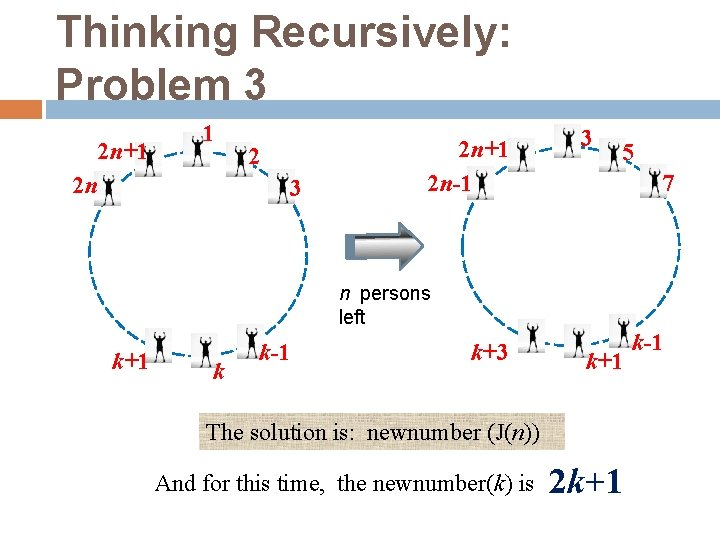Thinking Recursively: Problem 3 2 n+1 1 2 n 2 3 2 n+1 2 n-1 3 5 7 n persons left k+1 k k-1 k+3 k+1 The solution is: newnumber (J(n)) And for this time, the newnumber(k) is. . . 2 k+1 k-1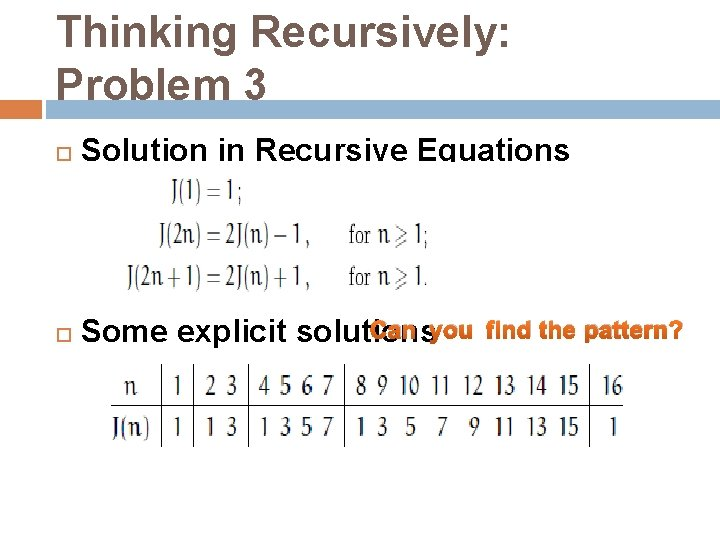Thinking Recursively: Problem 3 Solution in Recursive Equations Can you Some explicit solutions find the pattern?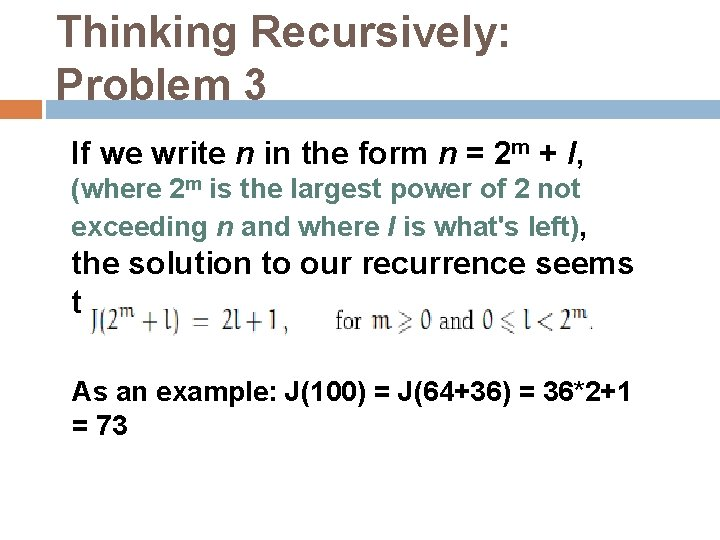Thinking Recursively: Problem 3 If we write n in the form n = 2 m + l, (where 2 m is the largest power of 2 not exceeding n and where l is what's left), the solution to our recurrence seems to be: As an example: J(100) = J(64+36) = 36*2+1 = 73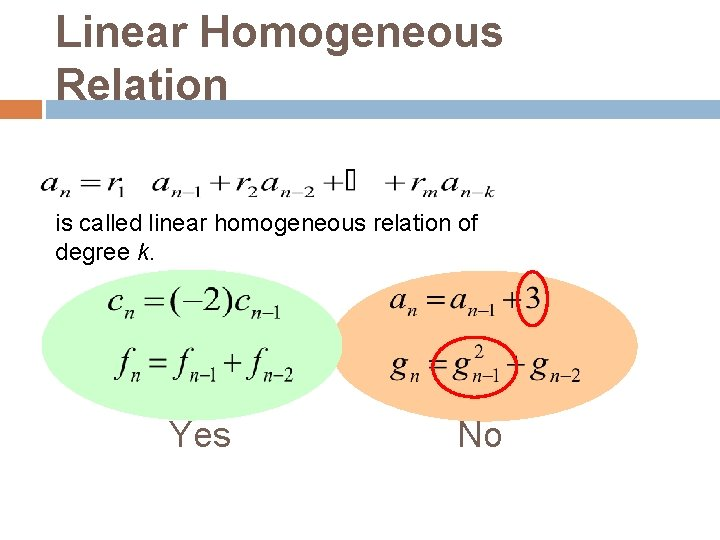Linear Homogeneous Relation is called linear homogeneous relation of degree k. Yes NoCharacteristic Equation For a linear homogeneous recurrence relation of degree k the polynomial of degree k is called its characteristic equation. The characteristic equation of linear homogeneous recurrence relation of degree 2 is: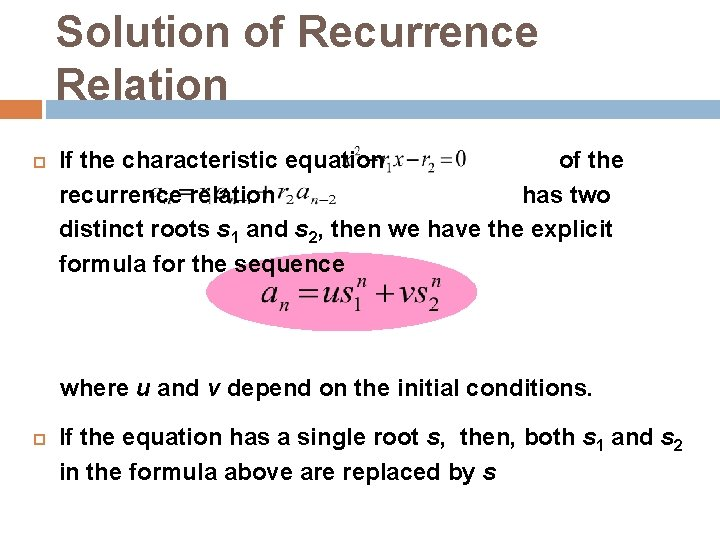Solution of Recurrence Relation If the characteristic equation of the recurrence relation has two distinct roots s 1 and s 2, then we have the explicit formula for the sequence where u and v depend on the initial conditions. If the equation has a single root s, then, both s 1 and s 2 in the formula above are replaced by sProof of the Solution the